# Changing Axis Labels in PowerPoint 2011 for Mac

Learn how to change the axis labels in PowerPoint 2011 for Mac.

Author:

Product/Version: PowerPoint 2011 for Mac

OS: Mac OS X

Axis Labels, as the name suggests are labels for the Categories and Values on your chart axes. Figure 1 below shows the default position of Axis Labels for a Column chart. Category names, which show as labels on the Horizontal (Category) axis have been highlighted in blue. Similarly Values show as labels on the Vertical (Values) axis -- these are highlighted in red.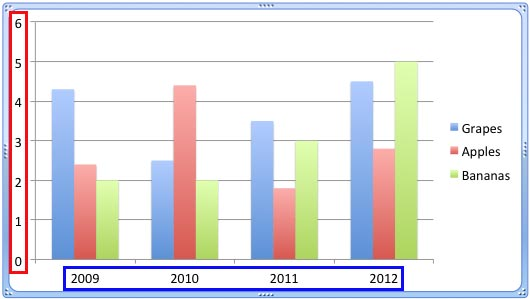Figure 1: Default Axis Labels in a Column chart

So where do these labels come from? Category Axis Labels reflect the Category names within your data (typically within an Excel sheet). Values on the other hand are determined by PowerPoint based upon the maximum value in your data -- the minimum value is always set to zero. Of course you can change the Minimum and Maximum values if you want.

Note: Values are typically shown as numbers or percentage values. They can also have decimals.
Tip: If you don't know how to insert a chart, refer to our Inserting Charts in PowerPoint 2011 for Mac tutorial. You can also change the chart type from one to another. Make sure your chart type supports axes.

Before you get started, you should have a chart inserted in your PowerPoint slide -- we use a Column chart for this example. Follow these steps to learn how you can change your Axis Labels -- let's start with changing Vertical (Value) Axis Labels:

## Changing Value Axis Labels

1. Select the Value Axis of the chart on your slide and right-click (or Ctrl+click) to access the context menu as shown in Figure 2. From the context menu, chose Format Axis option (refer to Figure 2 again).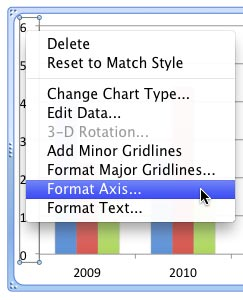Figure 2: Format Axis option selected for the Value Axis
2. This opens the Format Axis dialog box, as shown in Figure 3.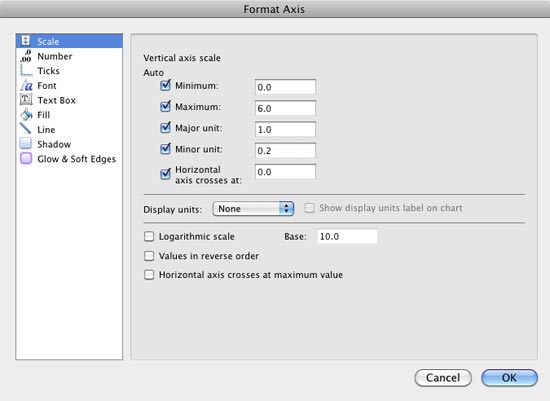Figure 3: Format Axis dialog box

In Figure 3 above, you can see all the Vertical axis' Scale options are set to default values -- we know so because the Auto check-box is selected for all these options. If you change the Maximum or Minimum value, or change the Major unit, you will see the Vertical Axis Labels reflecting the changes you made.

Look at Figure 4 where we have set the Maximum value to 10 (highlighted in red) -- the Vertical Axis Label automatically reflects this change, showing the Maximum value as 10 (highlighted in blue in Figure 4) -- compare this to the original chart shown in Figure 1.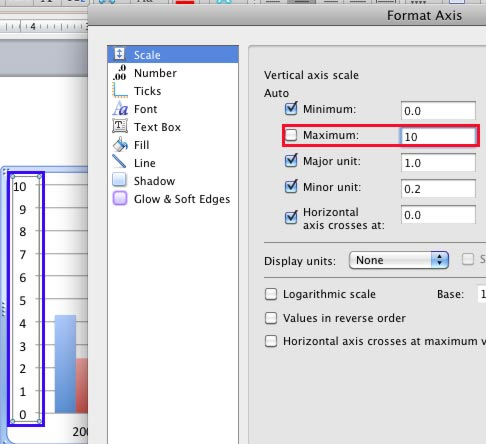Figure 4: Value Axis Label reflecting the changed Maximum value

Now, look at Figure 5 where we have changed the Display units to Hundreds (highlighted in red). Notice that the Vertical Axis Label now shows the changed display units (highlighted in blue within Figure 5).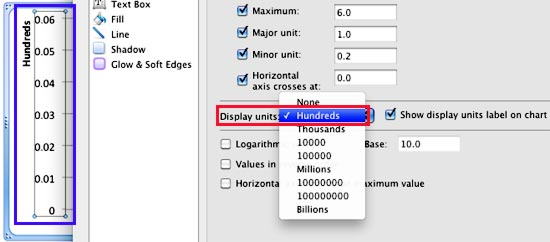Figure 5: Value Axis Label reflecting the changed Display units

You can also change the Value Axis Label to show as Currency. To do so, select the Number option in the sidebar (highlighted in green within Figure 6). Thereafter change the Category to Currency (highlighted in red within Figure 6) -- you can choose the Currency symbol you need, and also the decimal spaces. You can see that the resultant Value Axis Label (highlighted in blue in Figure 6) is now set to Currency.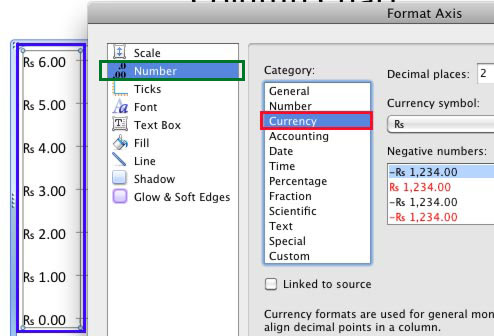Figure 6: Value Axis Label changed to Currency

You can similarly change the Value labels to show percentages, fractions, etc.

## Changing Category Axis Labels

Now, let us learn how to change the Category Axis Labels.

1. First select your chart -- then click the Edit button within the Charts tab of the Ribbon, as shown in Figure 7 (highlighted in red). This opens an instance of Excel with your chart data.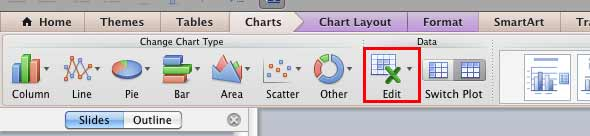Figure 7: Edit button within Data group
2. Change the Category names within the Excel sheet -- type new names for the Categories as required. Look at Figure 8 where we have changed our category names (highlighted in red).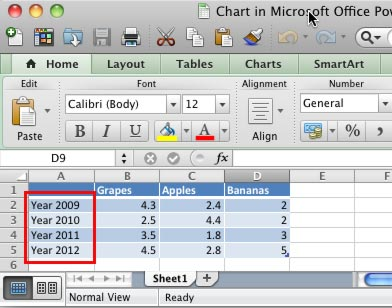Figure 8: Category names changed within the Excel sheet
3. Exit Excel -- the changes you make to the Category names in Excel will automatically be reflected in the Category Axis Labels on your chart within PowerPoint as shown in Figure 9 (highlighted in red) -- compare this to the original chart shown in Figure 1.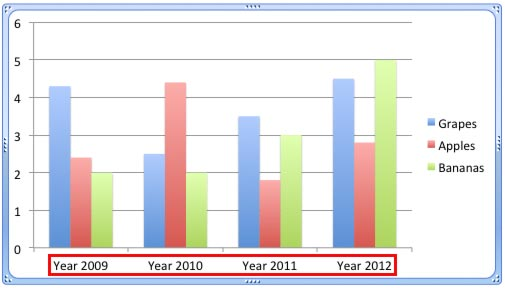Figure 8: Chart reflecting the changed category names